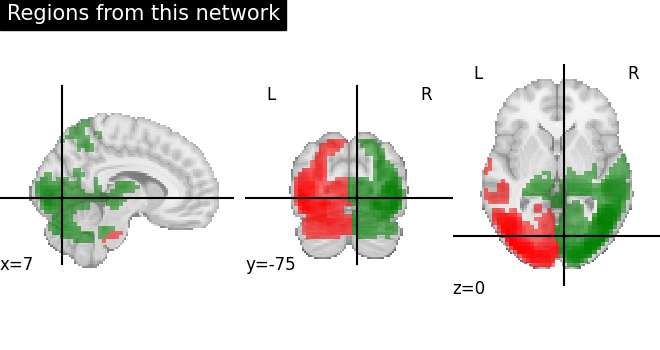# Regions extraction using dictionary learning and functional connectomes#

This example shows how to use `nilearn.regions.RegionExtractor` to extract spatially constrained brain regions from whole brain maps decomposed using Dictionary learning and use them to build a functional connectome.

We used 20 movie-watching functional datasets from `nilearn.datasets.fetch_development_fmri` and `nilearn.decomposition.DictLearning` for set of brain atlas maps.

This example can also be inspired to apply the same steps to even regions extraction using ICA maps. In that case, idea would be to replace Dictionary learning to canonical ICA decomposition using `nilearn.decomposition.CanICA`

Please see the related documentation of `nilearn.regions.RegionExtractor` for more details.

## Fetch brain development functional datasets#

```from nilearn import datasets

rest_dataset = datasets.fetch_development_fmri(n_subjects=20)
func_filenames = rest_dataset.func
confounds = rest_dataset.confounds
```

## Extract functional networks with Dictionary learning#

Import `DictLearning` from the `decomposition` module, instantiate the object, and `fit` the model to the functional datasets

```from nilearn.decomposition import DictLearning

# Initialize DictLearning object
dict_learn = DictLearning(
n_components=8,
smoothing_fwhm=6.0,
memory="nilearn_cache",
memory_level=2,
random_state=0,
standardize="zscore_sample",
)
# Fit to the data
dict_learn.fit(func_filenames)
# Resting state networks/maps in attribute `components_img_`
components_img = dict_learn.components_img_

# Visualization of functional networks
# Show networks using plotting utilities
from nilearn import plotting

plotting.plot_prob_atlas(
components_img,
view_type="filled_contours",
title="Dictionary Learning maps",
)
```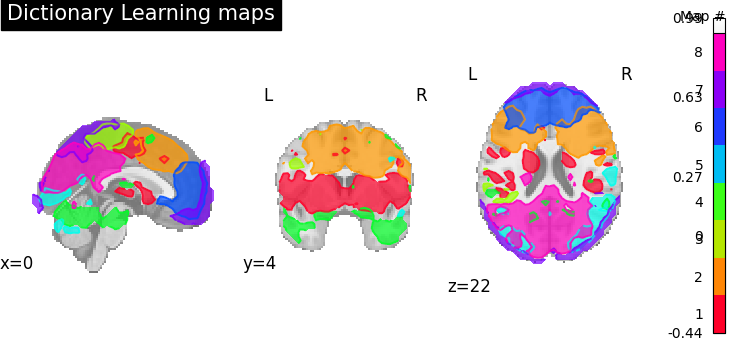```/opt/hostedtoolcache/Python/3.12.0/x64/lib/python3.12/site-packages/sklearn/decomposition/_dict_learning.py:658: FutureWarning:

'n_iter' is deprecated in version 1.1 and will be removed in version 1.4. Use 'max_iter' instead.

/opt/hostedtoolcache/Python/3.12.0/x64/lib/python3.12/site-packages/nilearn/plotting/displays/_axes.py:74: UserWarning:

linewidths is ignored by contourf

<nilearn.plotting.displays._slicers.OrthoSlicer object at 0x7f1ddbaa4320>
```

## Extract regions from networks#

Import `RegionExtractor` from the `regions` module. `threshold=0.5` indicates that we keep nominal of amount nonzero voxels across all maps, less the threshold means that more intense non-voxels will be survived.

```from nilearn.regions import RegionExtractor

extractor = RegionExtractor(
components_img,
threshold=0.5,
thresholding_strategy="ratio_n_voxels",
extractor="local_regions",
standardize="zscore_sample",
standardize_confounds="zscore_sample",
min_region_size=1350,
)
# Just call fit() to process for regions extraction
extractor.fit()
# Extracted regions are stored in regions_img_
regions_extracted_img = extractor.regions_img_
# Each region index is stored in index_
regions_index = extractor.index_
# Total number of regions extracted
n_regions_extracted = regions_extracted_img.shape[-1]

# Visualization of region extraction results
title = (
"%d regions are extracted from %d components."
"\nEach separate color of region indicates extracted region"
% (n_regions_extracted, 8)
)
plotting.plot_prob_atlas(
regions_extracted_img, view_type="filled_contours", title=title
)
```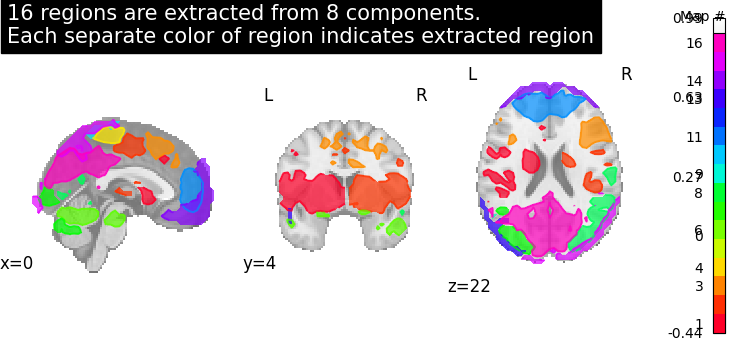```<nilearn.plotting.displays._slicers.OrthoSlicer object at 0x7f1df3c933b0>
```

## Compute correlation coefficients#

First we need to do subjects timeseries signals extraction and then estimating correlation matrices on those signals. To extract timeseries signals, we call `transform` onto each subject functional data stored in `func_filenames`. To estimate correlation matrices we import connectome utilities from nilearn.

```from nilearn.connectome import ConnectivityMeasure

correlations = []
# Initializing ConnectivityMeasure object with kind='correlation'
connectome_measure = ConnectivityMeasure(
kind="correlation",
standardize="zscore_sample",
)
for filename, confound in zip(func_filenames, confounds):
# call transform from RegionExtractor object to extract timeseries signals
timeseries_each_subject = extractor.transform(filename, confounds=confound)
# call fit_transform from ConnectivityMeasure object
correlation = connectome_measure.fit_transform([timeseries_each_subject])
# saving each subject correlation to correlations
correlations.append(correlation)

# Mean of all correlations
import numpy as np

mean_correlations = np.mean(correlations, axis=0).reshape(
n_regions_extracted, n_regions_extracted
)
```

## Plot resulting connectomes#

First we plot the mean of correlation matrices with `plot_matrix`, and we use `plot_connectome` to plot the connectome relations.

```title = f"Correlation between {int(n_regions_extracted)} regions"

# First plot the matrix
display = plotting.plot_matrix(
mean_correlations, vmax=1, vmin=-1, colorbar=True, title=title
)

# Then find the center of the regions and plot a connectome
regions_img = regions_extracted_img
coords_connectome = plotting.find_probabilistic_atlas_cut_coords(regions_img)

plotting.plot_connectome(
mean_correlations, coords_connectome, edge_threshold="90%", title=title
)
```
•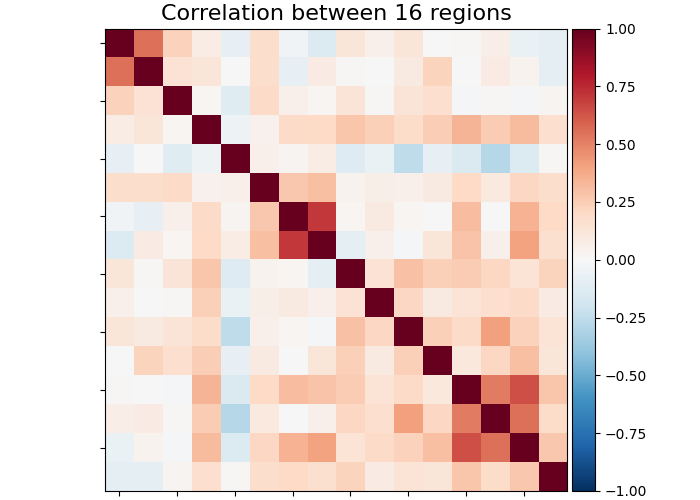•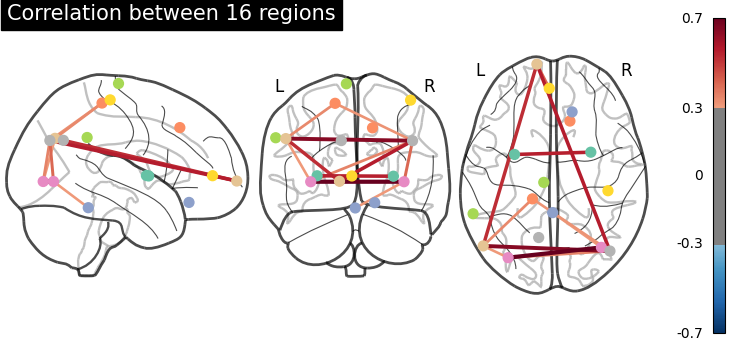```<nilearn.plotting.displays._projectors.OrthoProjector object at 0x7f1ddba7f440>
```

## Plot regions extracted for only one specific network#

First, we plot a network of `index=4` without region extraction (left plot).

```from nilearn import image

img = image.index_img(components_img, 4)
coords = plotting.find_xyz_cut_coords(img)
display = plotting.plot_stat_map(
img,
cut_coords=coords,
colorbar=False,
title="Showing one specific network",
)
```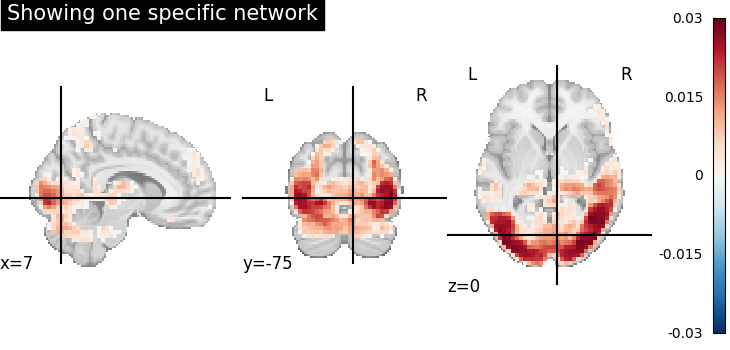Now, we plot (right side) same network after region extraction to show that connected regions are nicely separated. Each brain extracted region is identified as separate color.

For this, we take the indices of the all regions extracted related to original network given as 4.

```regions_indices_of_map3 = np.where(np.array(regions_index) == 4)

display = plotting.plot_anat(
cut_coords=coords, title="Regions from this network"
)

# Add as an overlay all the regions of index 4
colors = "rgbcmyk"
for each_index_of_map3, color in zip(regions_indices_of_map3, colors):